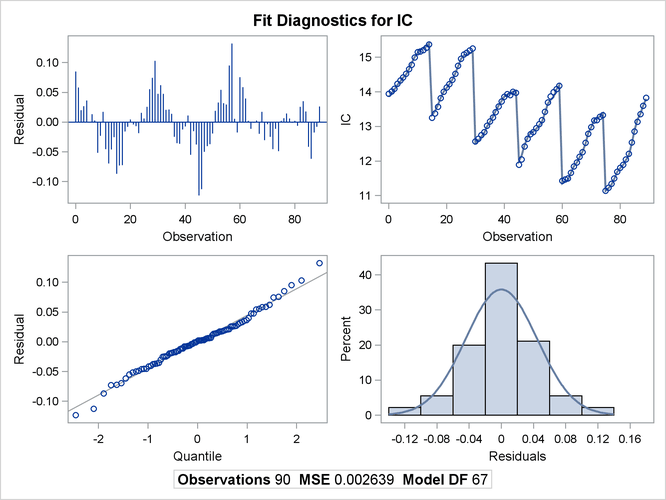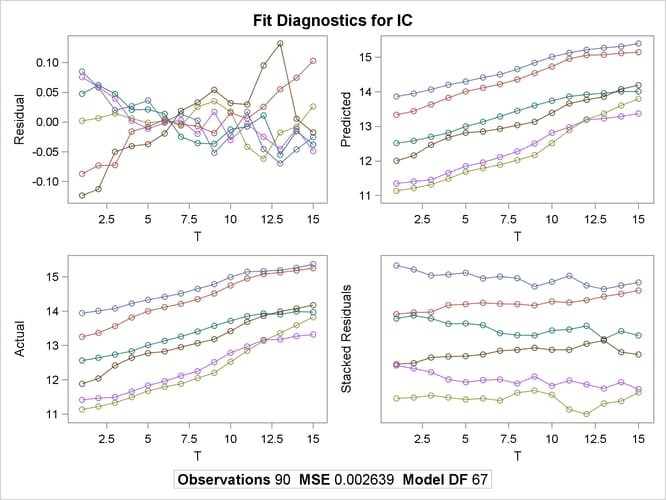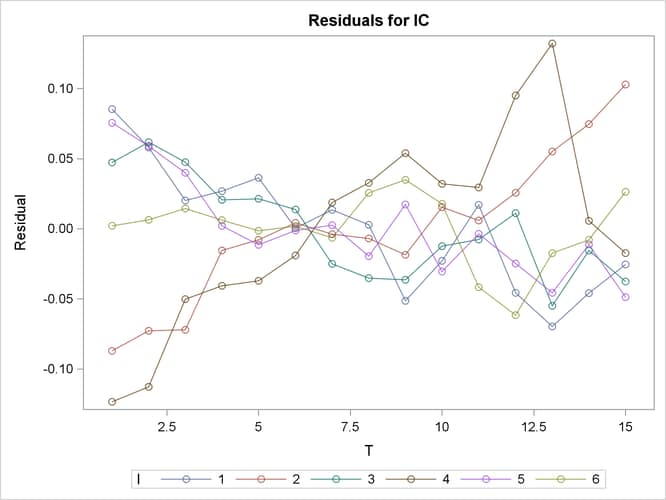The PANEL Procedure

ODS Graphics Plots

ODS graphics plots can be obtained to graphically analyze the results. The following statements show how to generate the plots. If the PLOTS=ALL option is specified, all available plots are produced in two panels. For a complete list of options, see the section Creating ODS Graphics.

```proc panel data=airline;
id i t;
model lC = lQ lPF LF / fixtwo plots = all;
run;
```

The preceding statements result in plots shown in Output 20.10 and Output 20.11.

Output 20.10: Diagnostic Panel 1Output 20.11: Diagnostic Panel 2The UNPACK and ONLY options produce individual detail images of paneled plots. The graph shown in Output 20.12 shows a detail plot of residuals by cross section. The packed version always puts all cross sections on one plot while the unpacked one shows the cross sections in groups of ten to avoid loss of detail.

```proc panel data=airline;
id i t;
model lC = lQ lPF LF / fixtwo plots(unpack only) = residsurface;
run;
```

Output 20.12: Surface Plot of the Residual